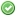rfc:alpanumeric_decrement

This is an old revision of the document!

# PHP RFC: Alphanumeric Decrement

## Introduction

This will add support for decrementing alphanumeric strings (“ab”, for example) to complement the existing incrementing behaviour.

## Proposal

At present, incrementing alphanumeric strings is supported. For example:

```\$x = "z";
\$x++;
// \$x is now "aa"```

However, decrementing alphanumeric strings is not:

```\$x = "z";
\$x--;
// \$x is still "z"```

Under this proposal, alphanumeric strings would be decrementable:

```\$x = "z";
\$x--;
// \$x is now "y"```

It would also handle the edge case of decrementing from “a”, choosing to result in 0 (this may be subject to change):

```\$x = "a";
\$x--;
// \$x is now 0```

The patch at present additionally changes how empty strings are incremented. At present, it works as follows:

```\$x = "";
\$x++;
// \$x is now "1" (string)
\$x = "";
\$x--;
// \$x is now -1 (int)```

With this patch, it is now consistent:

```\$x = "";
\$x--;
// \$x is now "-1" (string)```

## Backward Incompatible Changes

This might arguably be a BC break, as now decrementing for these strings would work, while existing code might assume that doesn't work. I doubt there is any code relying on that though, especially since “a”++ is a relatively obscure feature.

## Proposed PHP Version(s)

Next PHP 5.x. Failing that, next PHP x.

None.

None.

None.

None.

## Open Issues

How should decrementing “a” be handled? The patch and behaviour as proposed decrement to 0, however possibly not decrementing and emitting a warning might make more sense.

## Unaffected PHP Functionality

Does not affect \$x += 1 and \$x -= 1, nor \$x = \$x + 1 and \$x = \$x - 1, as both of these do not support alphanumeric increment at present.

## Future Scope

In future, alphanumeric increment being supported \$x += 1 and \$x = \$x + 1 might be desirable, but that is not within the scope of this RFC.

## Vote

Merge into PHP 5.6? (Will assume this requires 2/3 majority, due to new language feature)

Alphanumeric Decrement
Real name Yes No
ajf (ajf)chobieeee (chobieeee)datibbaw (datibbaw)daverandom (daverandom)derick (derick)dm (dm)dmitry (dmitry)dsp (dsp)krakjoe (krakjoe)laruence (laruence)levim (levim)lstrojny (lstrojny)malukenho (malukenho)peehaa (peehaa)philstu (philstu)pollita (pollita)shm (shm)stas (stas)treffynnon (treffynnon)tyrael (tyrael)willfitch (willfitch)Count: 0 21

## Patches and Tests

Considered final patch, unless bugs are found.

## Implementation

Not merged in at present.

## Rejected Features

None as yet?

### Page Tools﻿